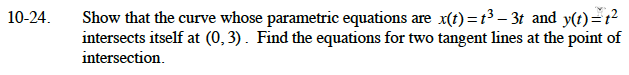### Home > CALC > Chapter Ch10 > Lesson 10.1.3 > Problem10-24

10-24.

Show that the curve whose parametric equations are x(t) = t3 −3t and y(t) = t2 intersects itself at (0, 3). Find the equations for two tangent lines at the point of intersection. Homework Help ✎Notice that y(t) = t2. At the point (0, 3), t2 = 3. How many solutions does this equation have?
What is the value of x(t) for these solutions?

To compute the slopes of the tangent lines at this point, use: